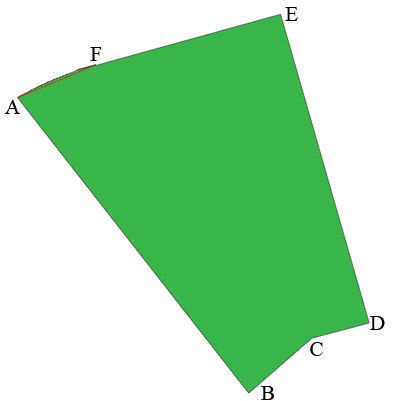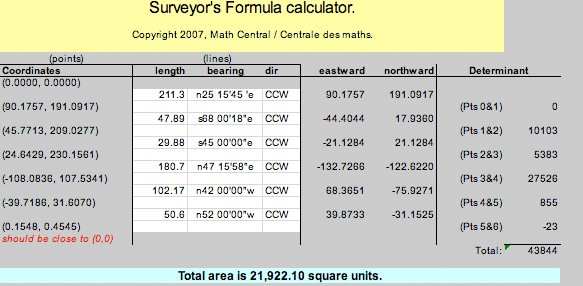SEARCH HOMEMath Central Quandaries & QueriesPlease help me find the area of my lot. I am sending a fax of the drawing and dimensions. ZackZack,

I redrew a diagram of the lot and labeled some points. I also extracted the dimensions.Dimensions
Side Length Bearing
AB 211.30 N 25 15' 58" E
BC 47.89 S 68 00" 18" E
CD 29.88 S 45 00' 00" E
DE 180.70 N 47 15' 58" E
EF 102.17 N 42 44' 02" W
FA 50.6 N 52 00' 00" W

The side FA is slightly curved So I approximated this side by the straight line from F to A and I approximated the bearing of this line in the table above.

I used the Surveyor's Area formula that Chris Fisher described in an answer to an earlier question and a Microsoft Excel tool the Steve La Rocque constructed to calculate the area.(You can download the spreadsheet in MS excel format if you like).

You diagram didn't give units but I expect the side lengths are given in feet. There are 43,560 square feet in an acre so my calculations give 21,922.10/43,560 = 0.50 acres.

I hope this helps,

HarleyMath Central is supported by the University of Regina and The Pacific Institute for the Mathematical Sciences.